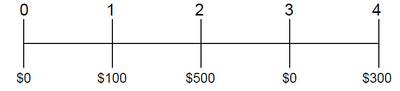#### Time Value of Money Overview

Money deposited in a bank account will earn interest income, so most people prefer to receive money today rather than the same amount in the future – this concept is referred to as “present discounted value”.

The required rate of return (aka discount rate) on investment is determined by three main factors:

Discount Rate = Risk Free Rate + Inflation Premium + Risk Premium

· Risk-Free Rate: the rate earned on a risk-less investment (U.S. Treasury Note)

· Inflation Premium: required return to account for the rise in the general level of prices for goods and services (purchasing power falls)

· Risk Premium: required return for being exposed to various types of investment risk; higher risk = higher risk premium; lower risk = lower risk premium

### Present Value

Present Value is today’s value of a cash flow that will be received in the future.

PV =   FV

(1 + r) N

PV = Present Value

FV = Future Value

r = Discount Rate

N = # of years

Example:

Given a 10% discount rate, calculate the present value of a \$100 cash flow that will be received in 3 years.

PV = \$100 = \$75.13

(1+.10)3

### Future Value

Future Value is the amount to which current cash flow will grow over a specific period at a specific interest rate:

FV = PV x (1 + i) N

PV = Present Value

FV = Future Value

i = interest rate

N = # of years

Example:

Calculate the FV of a \$250 investment at the end of 5 years if it earns an annual interest rate of 8%  FV = \$250 x (1+.08)5 = \$367.33

### Perpetuity

Perpetuity is a constant stream of identical cash flows over an infinite period of time. The formula for determining the present value of a perpetuity is as follows:

PV =  CF+ CF + CF + ….. = CF

(1+r)1(1+r)2 (1+r)3   r

PV = Present Value

CF = Cash Flow

r = Discount Rate

British console bonds and most preferred stocks are examples of perpetuities.

Example:

Assume that IBM’s preferred stock pays an annual dividend of \$5.00 and plans to continue this policy forever. Given an 8% discount rate, what is the value of IBM's preferred stock?

PV = \$5.00/8% = \$62.50#### PV and FV of Uneven Cash Flow Series

It is common to evaluate cash flow streams that are not equal from period to period.

Example:

Calculate the present and future values given a 10% discount rate:

PV = \$0 + \$100 + \$500 + \$0+ \$300 = \$709.03

(1+.10)0(1+.10)1 (1+.10)2 (1+.10)3 (1+.10)4

FV = (\$0 x 1.14) + (\$100 x 1.13) + (\$500 x 1.12) + (\$0 x 1.11) + (\$300 x 1.10) = \$1,038.10